# Graphing Calculator Drawing: Lissajous Curves

This post is part of a series.

Demonstration - Lissajous Curves. Lissajous curves take the form

\begin{align*} x &= \sin(t) \\[5pt] y &= \sin(at+b) \end{align*}

for some values of $a$ and $b.$ Observe the graph as you type each of the following Lissajous plot inputs, with $0 \leq t \leq 100.$

\begin{align*} &\left( \sin(t), \sin(t+1) \right) \\[10pt] &\left( \sin(t), \sin(t+2) \right) \\[10pt] &\left( \sin(t), \sin(t+3) \right) \\[10pt] &\left( \sin(t), \sin(2t+1) \right) \\[10pt] &\left( \sin(t), \sin(3t+1) \right) \\[10pt] &\left( \sin(t), \sin(4t+1) \right) \\[10pt] &\left( \sin(t), \sin(5t+1) \right) \\[10pt] &\left( \sin(t), \sin(1.1t+1) \right) \\[10pt] &\left( \sin(t), \sin(1.2t+1) \right) \\[10pt] &\left( \sin(t), \sin(1.3t+1) \right) \\[10pt] &\left( \sin(t), \sin(1.4t+1) \right) \\[10pt] &\left( \sin(t), \sin(1.5t+1) \right) \end{align*}

Exercise. Attempt to reproduce the graphs below by setting $a=1$ and varying the $b$ parameter in the Lissajous curve equations. You may have to play with the parameter a bit to get a sense of what it controls.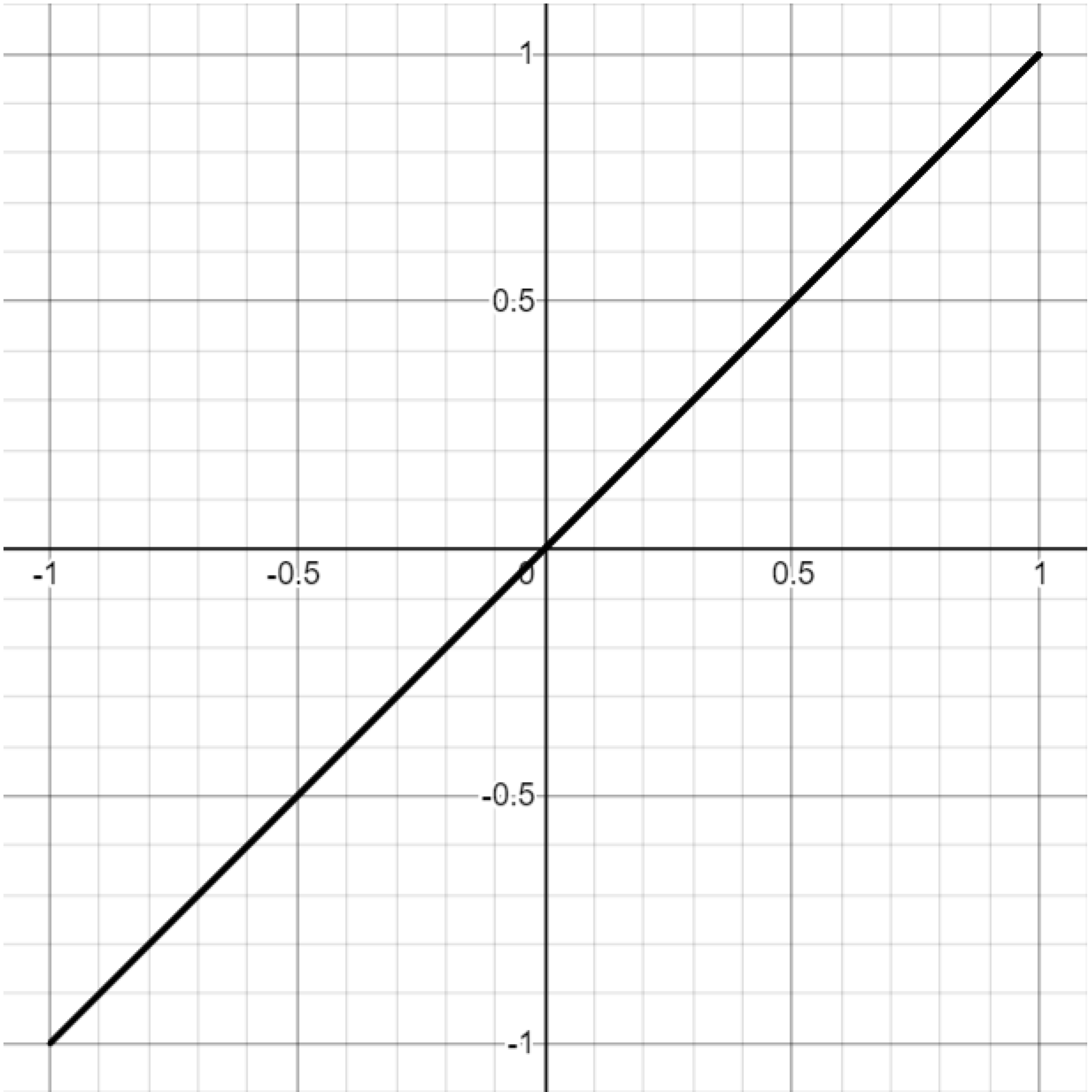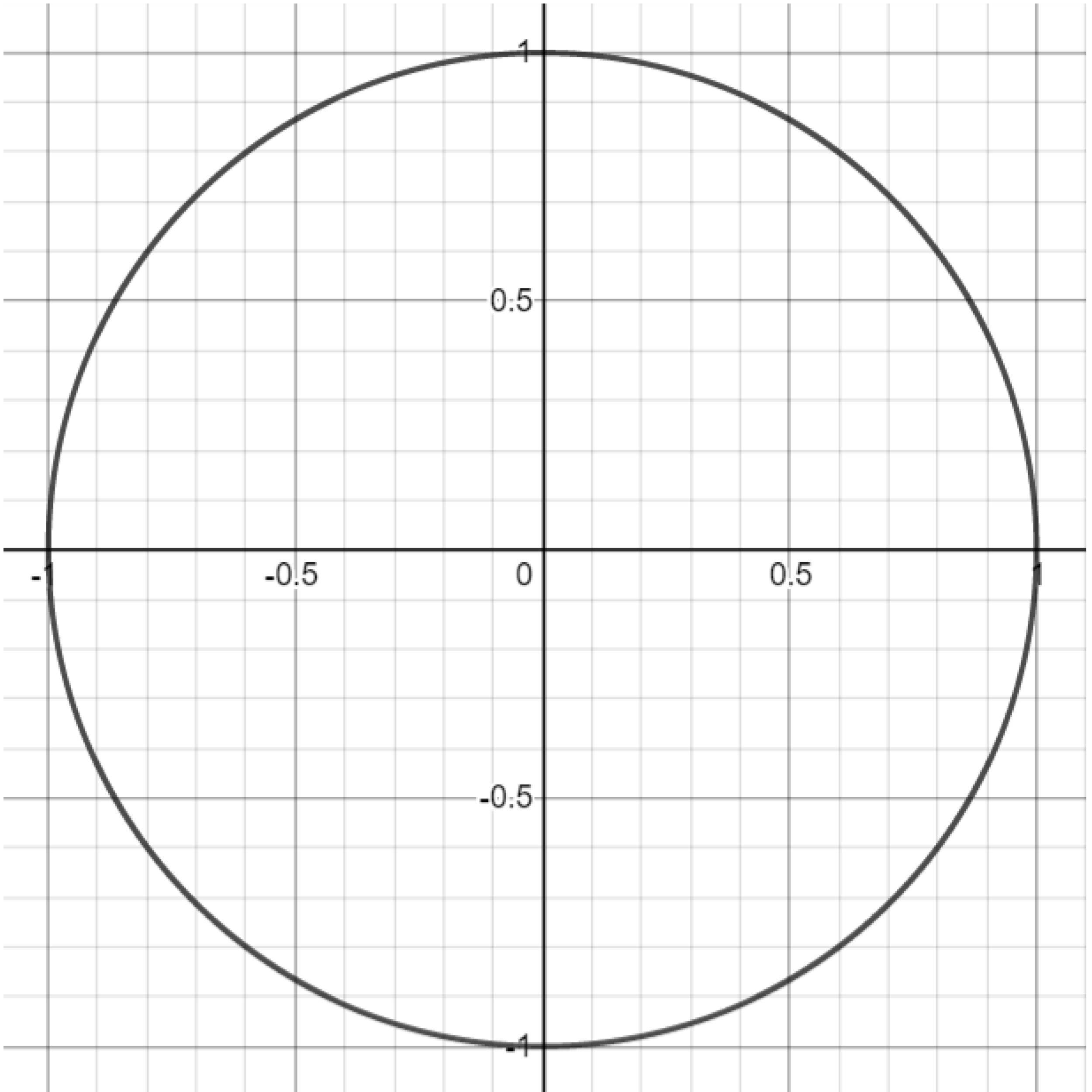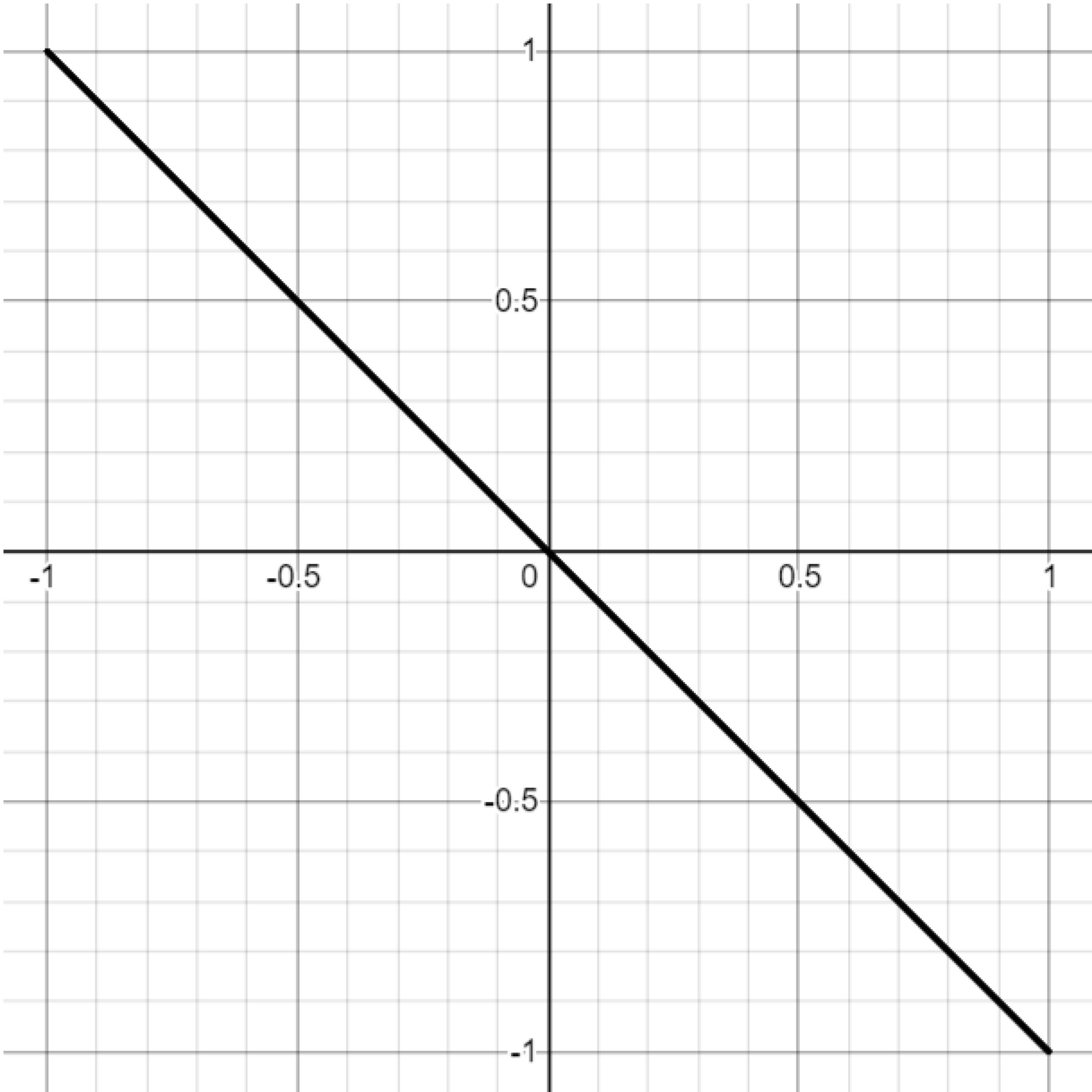Exercise. Attempt to reproduce the graphs below by setting $b=\frac{\pi}{2}$ and varying the $a$ parameter in the Lissajous curve equations. You may have to play with the parameter a bit to get a sense of what it controls.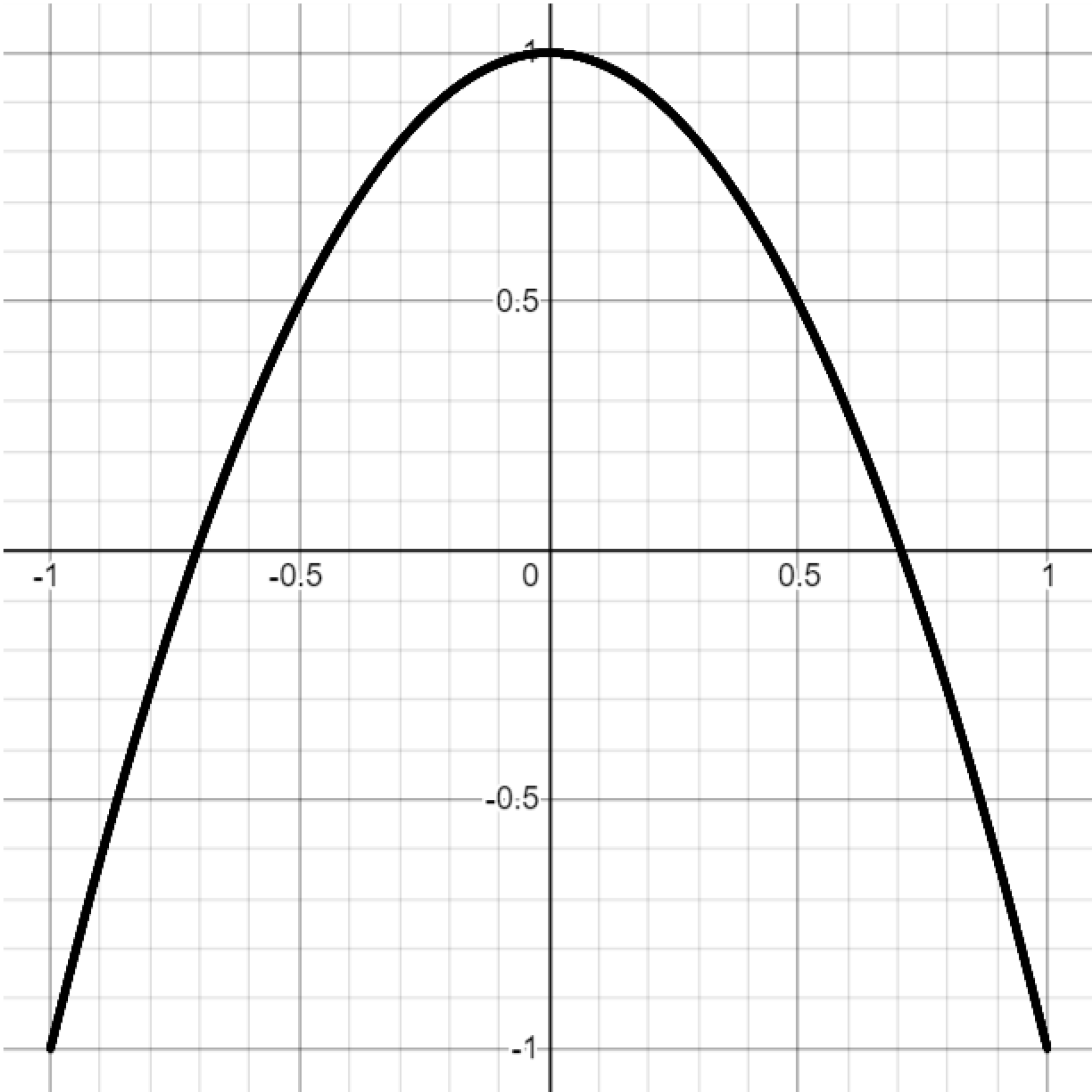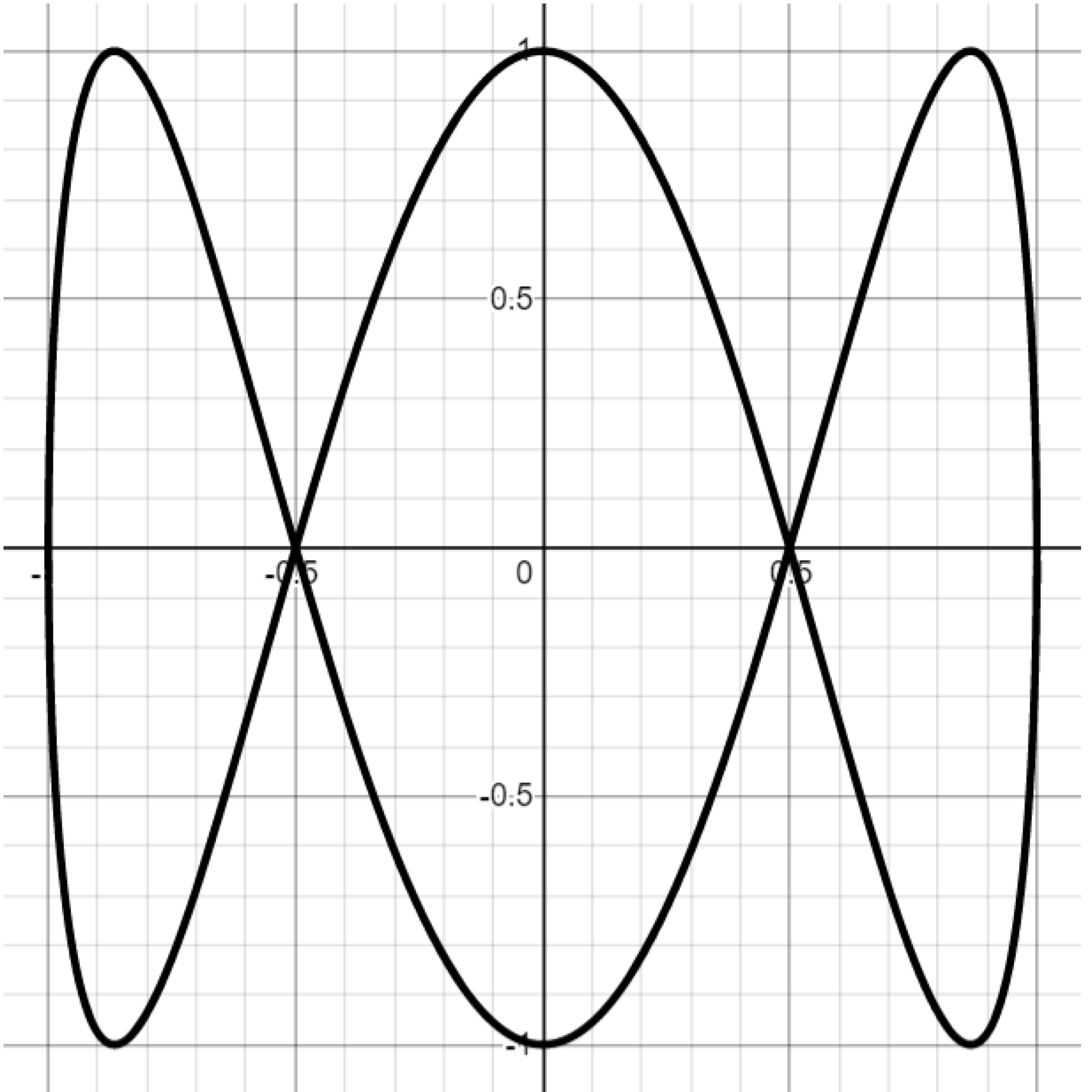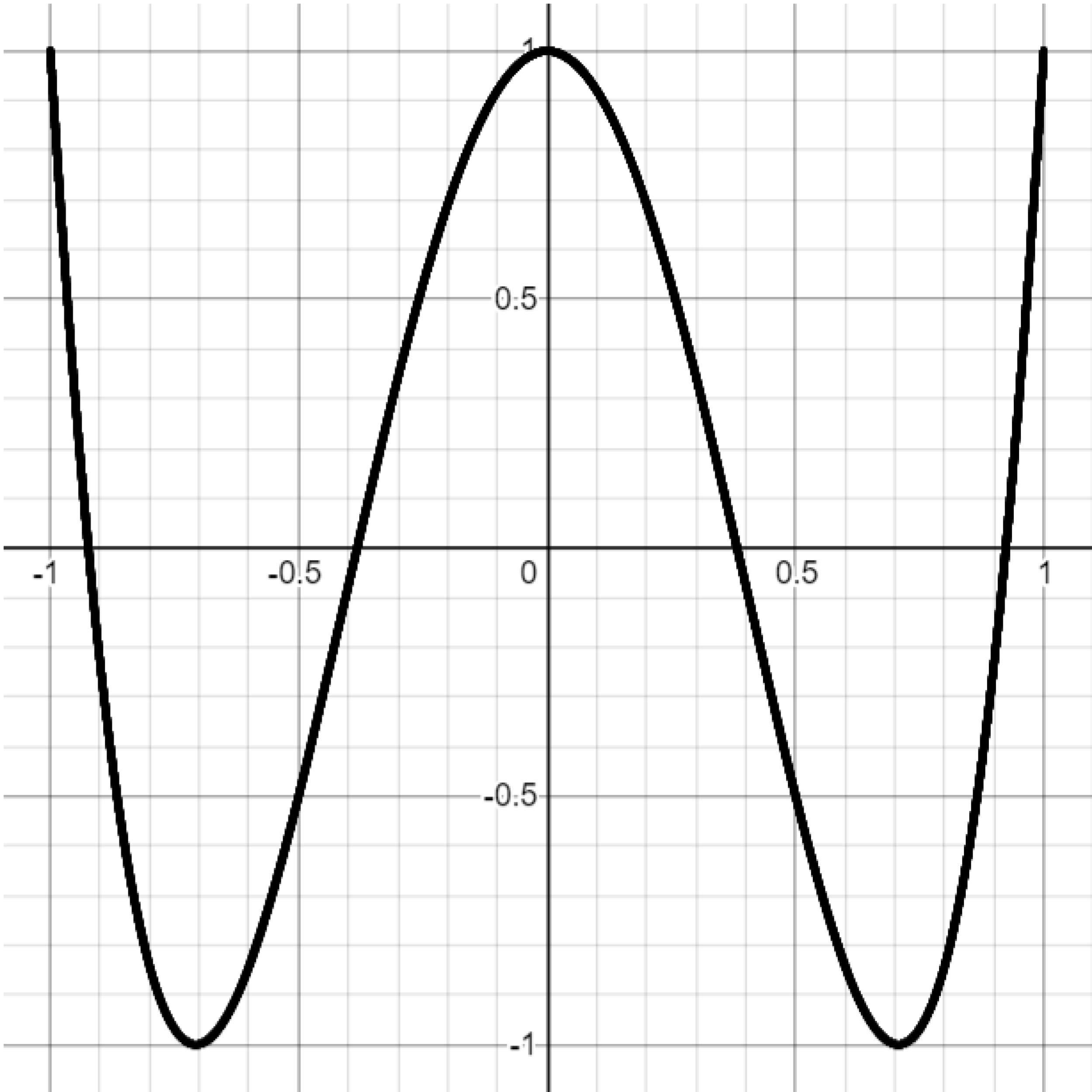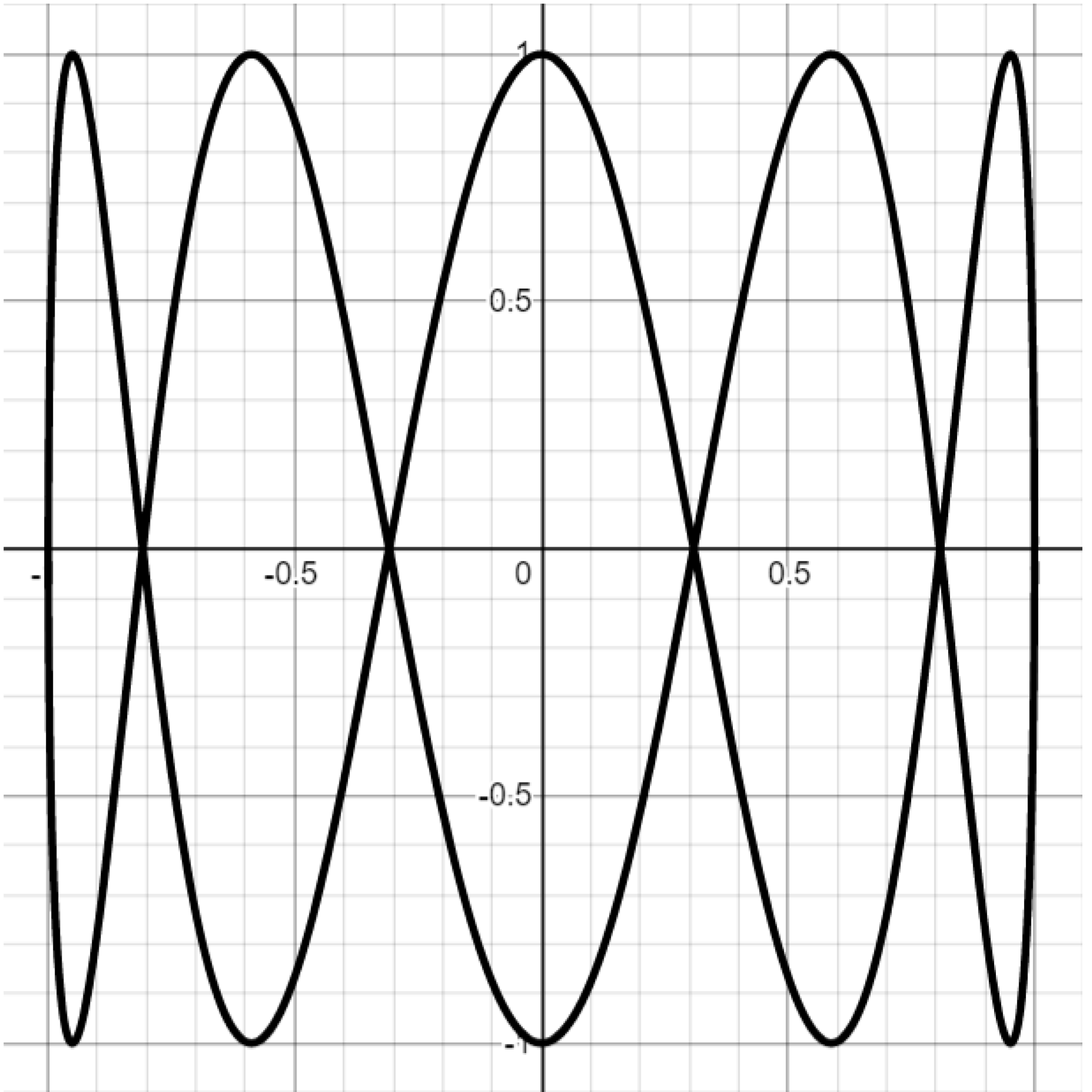Challenge. Attempt to reproduce the Lissajous graphs below by setting $b=1$ and varying $a.$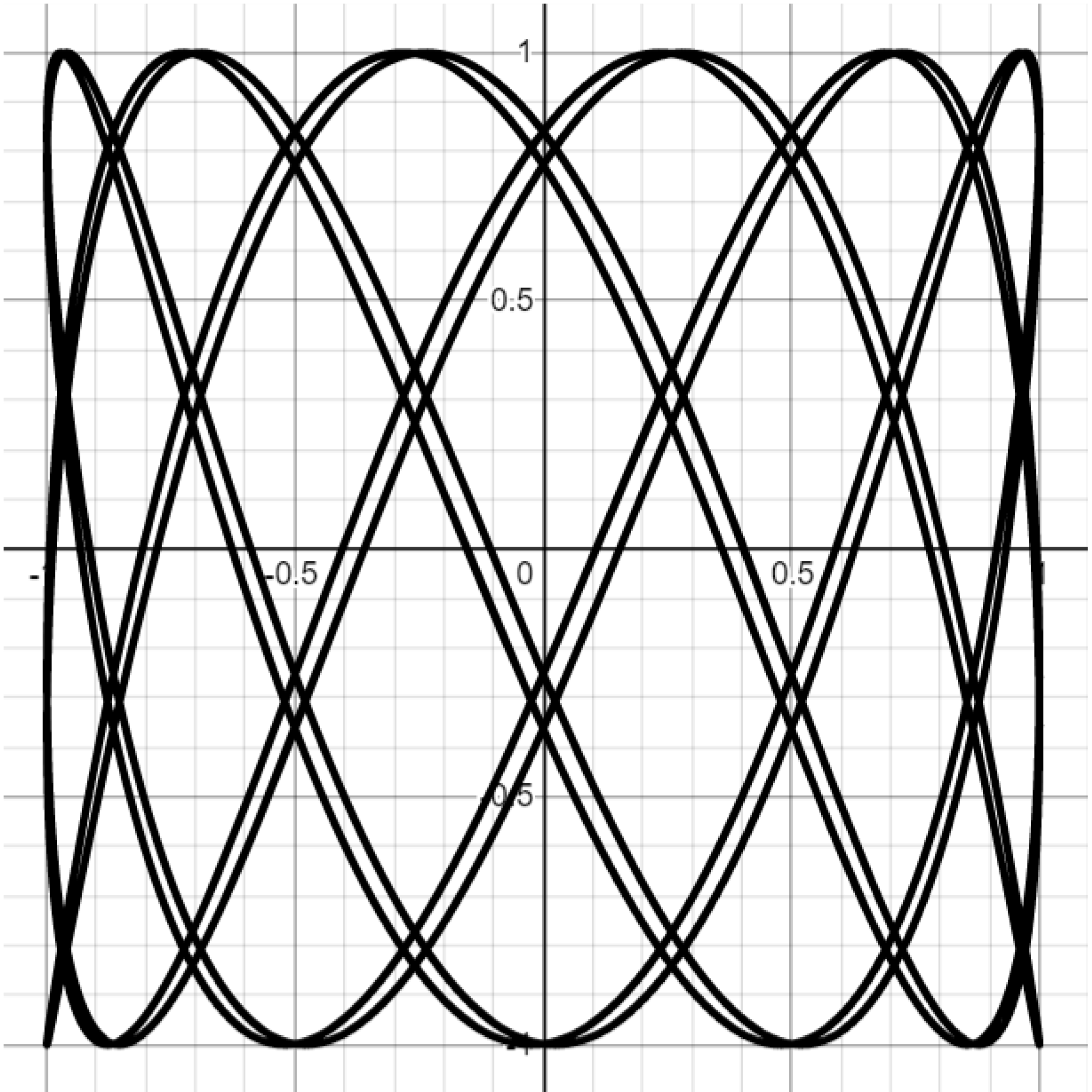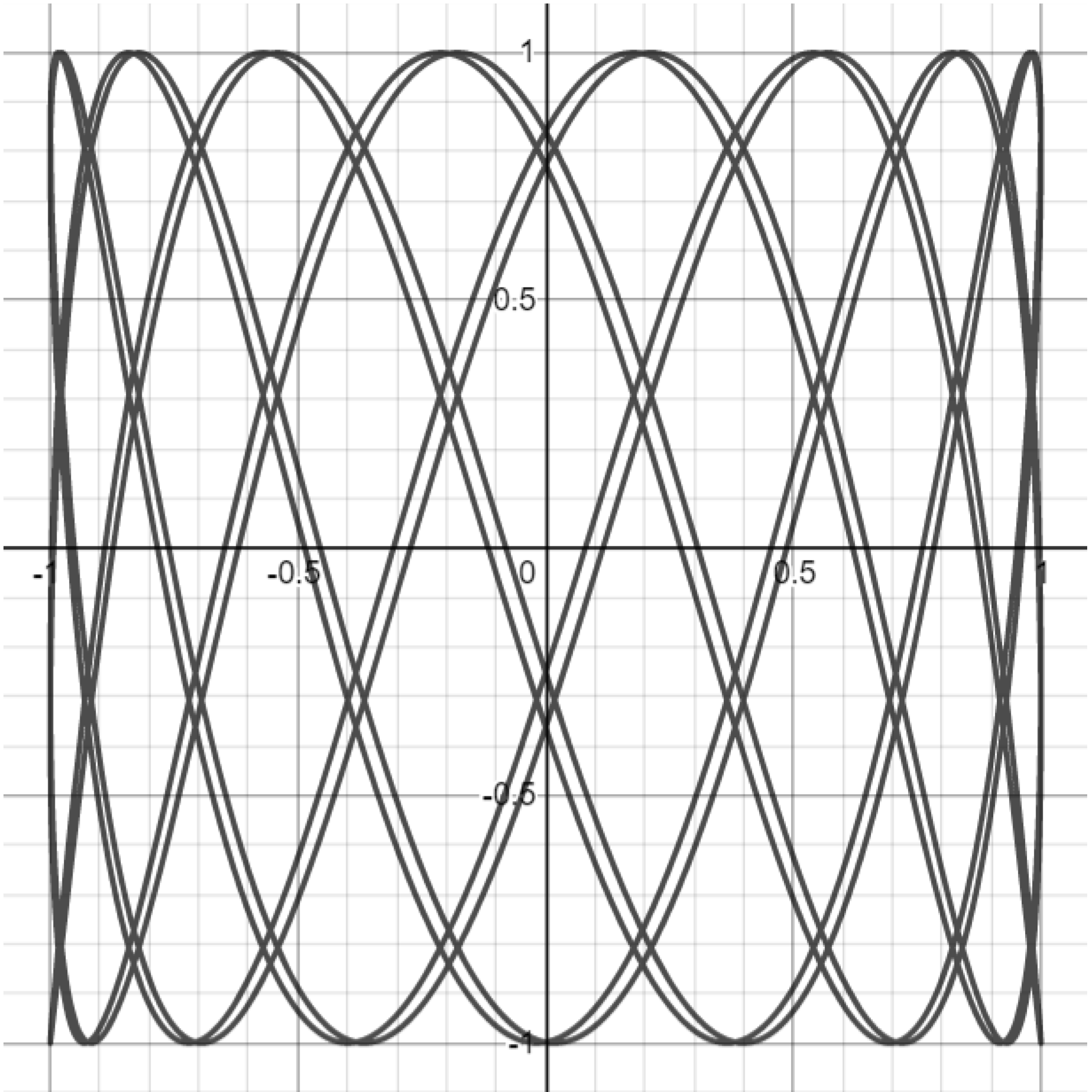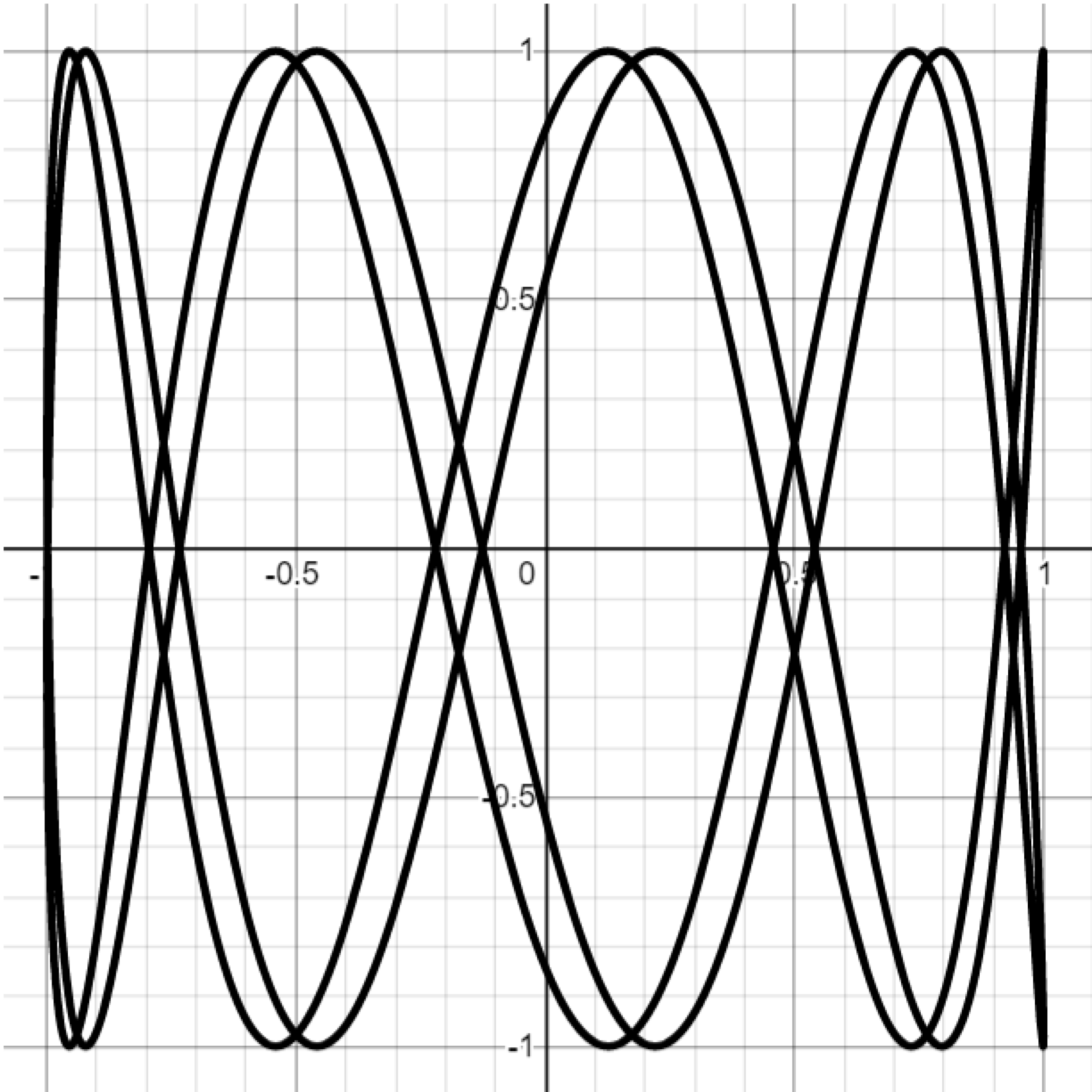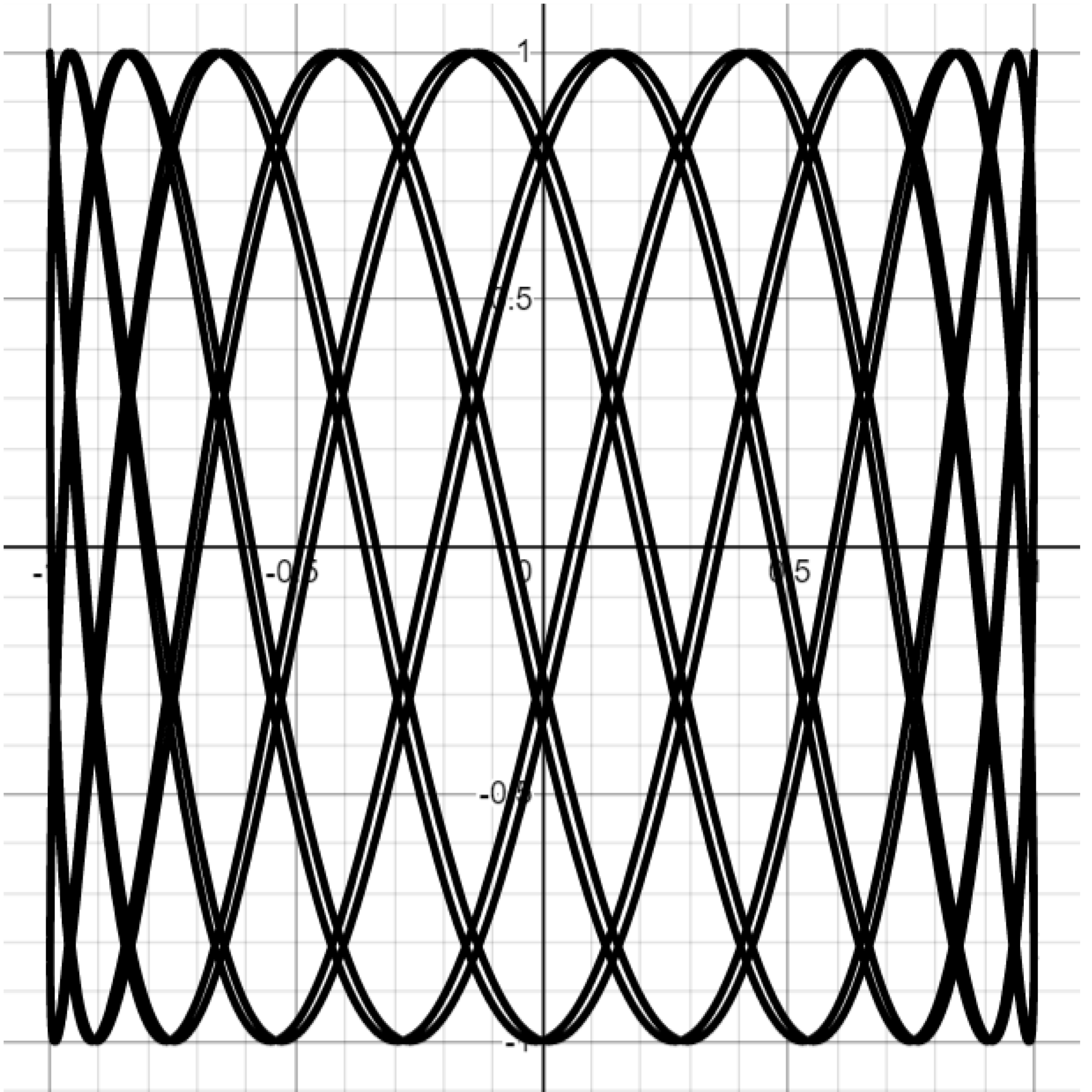This post is part of a series.

Tags: